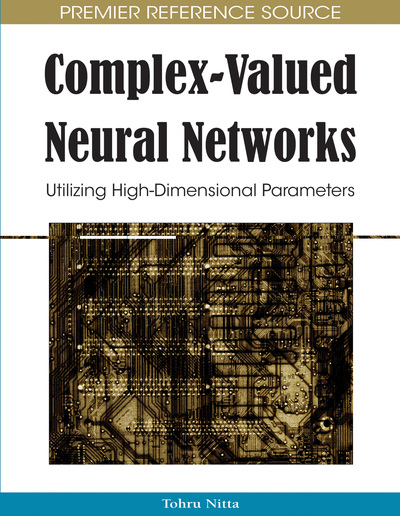Shigeo Sato, Mitsunaga Kinjo
DOI: 10.4018/978-1-60566-214-5.ch014
OnDemand:
(Individual Chapters)
Available
\$37.50
No Current Special Offers

## Abstract

The advantage of quantum mechanical dynamics in information processing has attracted much interest, and dedicated studies on quantum computation algorithms indicate that a quantum computer has remarkable computational power in certain tasks. Quantum properties such as quantum superposition and quantum tunneling are worth studying because they may overcome the weakness of gradient descent method in classical neural networks. Also, the technique established for neural networks can be useful for developing a quantum algorithm. In this chapter, first the authors show the effectiveness of incorporating quantum dynamics and then propose neuromorphic adiabatic quantum computation algorithm based on the adiabatic change of Hamiltonian. The proposed method can be viewed as one of complex-valued neural networks because a qubit operates like a neuron. Next, the performance of the proposed algorithm is studied by applying it to a combinatorial optimization problem. Finally, they discuss the learning ability and hardware implementation.
Chapter Preview
Top

## Introduction

Real parallel computing is possible with a quantum computer which makes use of quantum states (Nielsen & Chuang, 2000). It is well known that a quantum computation algorithm for factorization discovered by Shor (1994) is faster than any classical algorithm. Also a quantum algorithm for database search inquantum computation steps instead ofclassical steps has been proposed by Grover (1996). These algorithms are often referred to as representative quantum algorithms utilizing features of quantum dynamics. A quantum algorithm not limited to a specific problem is needed for the development of a quantum computer from the practical viewpoint. Farhi et al. have proposed an adiabatic quantum computation (AQC) for solving the three-satisfiability (3-SAT) problem (Farhi, Goldstone, Gutmann, & Sipser, 2000). AQC can be applied to various problems including non-deterministic polynomial time problems (NP Problems) if one can obtain proper Hamiltonians. A few methods have been proposed such as the original AQC by Farhi et al. (Farhi & Gutmann, 1996; Farhi et al., 2000; Farhi et al., 2001), AQCs utilizing analogy to quantum circuits (Aharonov et al., 2004; Siu, 2005), and neuromorphic AQC(Sato, Kinjo, & Nakajima 2003; Kinjo, Sato, Nakamiya, & Nakajima, 2005).

Neural networks can be applied to such combinatorial optimization problems. The most popular approach is to utilize a Hopfield neural network (HNN) (Hopfield, 1982). However, it has been known that an HNN is often trapped in local minima, and an optimal solution is not obtained in this case. This is because the dynamics of the network is determined by the local features of the energy potential. On the other hand, if we can incorporate quantum dynamics into a neural network, such undesired behavior could be avoided.

Many studies inspired by quantum computation or quantum mechanics have been reported in order to improve the performance of neural networks related to associative memories, learning ability, and so on (Ventura & Martinez, 2000; Ezhov & Ventura, 2000; Matsui, Takai, & Nishimura 1998, 2000; Peruš, Bischof, Caulfield, & Loo, 2004; Mori, Isokawa, Kouda, Matsui, & Nishimura, 2006). Neural networks having quantum properties can be viewed as one of complex-valued neural networks since its neuron state is expressed in complex numbers. Good introductions on quantum neural networks (QNN) are found in some chapters of this book. Also the study described in this chapter is related to QNN, and the research purpose is to introduce methods used in conventional neural networks to quantum computation. We focus on AQC inspired from an HNN. First the advantages of quantum dynamics and quantum computation are described together with simple examples. Next, neuromorphic adiabatic quantum computation (NAQC) is proposed by relating AQC with HNN in order to incorporate neuromorphic techniques to quantum computation. The performance of NAQC is evaluated when it is applied to the N-queen problem, which is one of the NP problems. The effect of energy dissipation is studied for favorable state transitions to lower energy states resulting in performance enhancement. Then, a learning method in NAQC is studied for practical use. Finally, hardware implementation of the proposed method is discussed.

## Complete Chapter List

Search this Book:
Reset